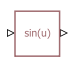# PS Math Function

Apply mathematical function to input physical signal

• Library:
• Simscape / Foundation Library / Physical Signals / Functions

•## Description

The PS Math Function block applies a mathematical function to the value and unit of the input physical signal, u. The block output is the result of the operation of the function on the input. You can select one of the following functions from the Function choice parameter list.

FunctionDescriptionMathematical Expression
`sin(u)`Sinesin(u)
`cos(u)`Cosinecos(u)
`exp(u)`Exponentialeu
`log(u)`Natural logarithmln(u)
`10.^u`Power of base 1010u
`log10(u)`Common (base 10) logarithmlog(u)
`u.^2`Power 2u2
`sqrt(u)`Square rootu0.5
`1./u`Reciprocal1/u
`tanh(u)`Hyperbolic tangenttanh(u)
`u.^v`Poweruv

The PS Math Function block issues a simulation-time error when the input falls out of the expected domain for the particular function used. For example, if set to `sqrt(u)`, the PS Math Function block issues an error if it receives negative input during simulation.

Note

For `u.^2`, `sqrt(u)`, `1./u`, and `u.^v` the unit of the output signal is the result of the operation of the function on the input signal unit. Thus for `u.^v`, unless the input signal is unitless, changing the value of v changes the unit of the output signal. For all other functions in the list, the input signal must be unitless.

## Ports

### Input

expand all

Input port for the operand physical signal, u.

The port name is not visible in the block icon, but you can see this name in the underlying source file by clicking the Source code link in the Description tab of the block dialog box.

### Output

expand all

Output physical signal.

The port name is not visible in the block icon, but you can see this name in the underlying source file by clicking the Source code link in the Description tab of the block dialog box.

## Parameters

expand all

Select the function to perform. The block output is the result of the operation of the function on the value and unit of the input signal.

For the `u.^v` option, the value of the output signal is the value of the input signal, `u`, to the power of v. If v is a vector or matrix, its size must match the size of the input signal, `u`, or `u` must be a scalar.

The unit of the output signal is the result of the operation of the function on the input signal unit. Therefore, unless the input signal is unitless, changing the value of v changes the unit of the output signal.

#### Dependencies

To enable this parameter, set Function choice to `u.^v`.

## Version History

Introduced in R2007b

expand all